#### Waves at the boundary of two media, Physics tutorial

The concept of wavefront and Huygens' construction:

Let us consider propagation of the wave on surface of water. If you dip finger in water constantly, the series of crests and troughs travel out. That is, waves set out in every direction. At any instant, the trough or crest is circular in shape. Locus of points in same phase at the particular time is known as a wavefront. Shape of wavefront depends on nature of source. In case of waves from the point source in air, wavefronts are spherical. If source is the long slit, wavefront will be cylindrical. At large distances from source whether point or slit), wavefront appears to be the plane. To understand formation of wavefronts, we utilize Huygens construction.

Following Huygens, we make the given assumptions:

i) Each point on the wavefront becomes the fresh source of secondary wavelets, that move out in all directions with speed of wave in that medium.

ii) New wavefront, at any later time, is given by forward envelope of secondary wavelets at that time.

iii) In the isotropic medium, energy carried by waves is transmitted equally in all directions.

According to Huygens's construction, in the figure given below surface AB is known as a primary wavefront. Each point on AB, like a, b, c, etc., serves as secondary source (derived from original source S). These secondary sources give out waves (or disturbances) in all directions as shown by drawing circles around points a, b, c, etc. The envelope of all these waves (which acts as the tangent to all of them at any given instant) like one at CD, forms another wavefront, known as secondary wavefront. This, in short, signifies that source S gives out wavelets in all directions. Envelope of the wavelets serves as the primary wavefront. Every point on this primary wavefront serves as source for secondary wavelets. The envelope of the secondary wavelets forms to visualize Huygens' construction in space; you may imagine the point source to be at centre of a hollow sphere. Outer surface of this sphere then serves as primary wavefront. If this sphere is further surrounded by another hollow sphere of larger radius, outer surface of second hollow sphere will then serves a secondary wavefront. If this sphere is further surrounded by another sphere of still bigger radius, surface of outermost sphere becomes the secondary wavefront. For this, surface of inner sphere serves as primary wavefront. In two dimensions, primary and secondary wavefronts seem to be concentric circles, parts of which are shown in figure given below.

Formation of secondary sources as visualized by Huygens can also be understood pictorially through a simple diagram. If we place the screen XY with small hole at S' in path of waves emanating from source S, S' serves as secondary source. This gives out waves on other side of the screen. These waves spread out from S' as if S' is the original source itself. We view these whenever the wave travelling in one medium, say air, meets boundary of another medium. Assume we clamp one end of the string to rigid wall and produce a pulse by moving the other end. You will observe that pulse is reflected at the fixed end. Likewise, you can study reflection of ripples in the water basin.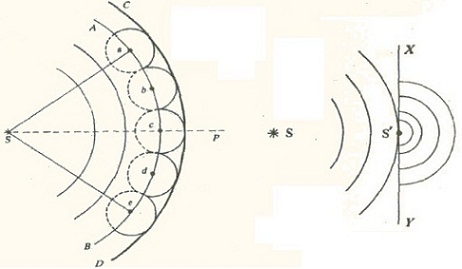Reflection of Waves:

In figure given below LM represents the part of the plane wavefront travelling towards the smooth reflecting surface S1S2, It first strikes at A and then at successive points towards D. If v is wave speed, point M on wavefront reaches D at time t = DC/ v later compared to the point L. According to Huygens' Principle, every point on reflecting surface will give rise to secondary wavelets. In this situation we expect that they should comprise reflected wavefront.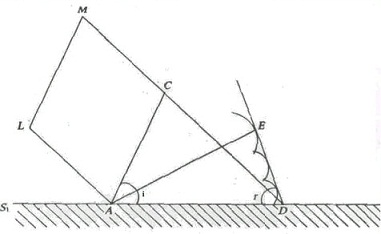The instant D is just disturbed; the wavelet from A has grown for time DC/ v and has travelled to E so that the distance AE is equal to DC. We can draw a circle of radius AE (= DC) to represent this wavelet with A as centre. Similarly, we can draw many circles from the intermediate points. Tangent or envelope to these circles from D states reflected wavefront. It is clear that Δs ACD and DEA, are congruent. Hence

or ∠i = ∠r..............................Eq.1

That is, angle of incidence is equal to angle of reflection. Furthermore, you will note that incident ray, reflected ray and normal at point of incidence lie in plane of the paper.

Refraction of Waves:

When the wave reaches boundary of two different media, it may be partially reflected and partially transmitted. Joining two strings: one thick and another thin so that their mass per unit lengths is different. The velocity of the wave is inversely proportional to density of medium. This signifies that when the wave moves from lighter to the denser medium, its velocity decreases. This results in the change (decrease) in wavelength. But frequency stays same.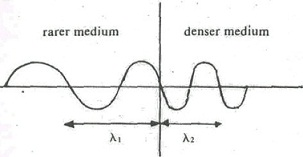λ1 > λ2

v1 > v2

Let vt and λt respectively indicate speed and wavelength of the wave of frequency f in rarer medium. On being refracted at interface of the denser medium, let its speed and wavelength be vd and λd, respectively. Mathematically, we can connect the quantities through relation

vtt = vd/λd..............................Eq.2

As f is same. This relation holds for waves in water, air and string alike.

Reflection and transmission amplitude coefficients:

Transverse Waves: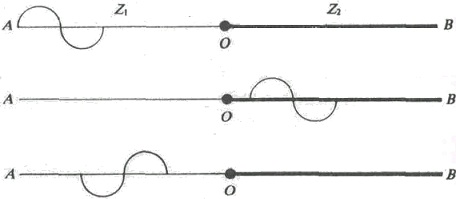Let us reconsider strings AO and OB joined together at O and kept under same tension T. Let us suppose that they provide characteristic impedances of Z1 and Z2, respectively. The wave travelling in positive x -direction as shown in figure gets partly reflected and partly transmitted at O. Particle displacements because of incident, reflected and transmitted waves can be written as:

yi(x,t) = aisin(ω0t - k1x) ..............................Eq.3

yr(x,t) = arsin(ω0t - k1x) ..............................Eq.4

and

yt(x,t) = atsin(ω0t - k2x) ..............................Eq.5

Where subscripts i , r and t on displacements and amplitudes refer to incident, reflected and transmitted waves, respectively. Angular frequency of these waves stays same. Furthermore propagation constant for incident and reflected waves is same but varies for transmitted wave. This is due to wave speed changes as density of medium changes. You would also note that for reflected wave we have utilized positive sign before k1x. This is due to it is travelling in negative x -direction.

Here total displacement and total transverse component of tension on one side of boundary are result of combination of incident and reflected waves. So boundary conditions in this situation are:

1) Particle displacements immediately to left and right of boundary (i.e. at x = 0 should also be same. This signifies that particle velocities ∂y(x,t)/∂t must also be same.

2) Transverse components of tension (-T(∂y(x,t)/∂x) should also be same immediately on two sides of boundary.

These conditions require:

yi(x,t)|x=0 + yr(x,t)|x=0 = yt(x,t)|x=0..............................Eq.6

And -T(∂yi/∂x)|x=0 + T∂yr/∂x|x=0 = -T∂yt/∂x|x=0..............................Eq.7

Condition defined by Eq.7 provides:

k1T(ai - ar) = k2Tat..............................Eq.8

Likewise, we can write

k2T = 2πfZ2

Where Z2 is impedance offered by second medium.

Using the results, we can rewrite Eq.8 as

Z1(ai - ar) = Z2at..............................Eq.9

Equations allow us to compute ratios ar/ai and at/ai. These ratios provide us fractions of incident amplitude reflected and transmitted at boundary. These ratios are generally known as reflection and transmission amplitude coefficients. We will indicate these by symbols R12 and T12:

R12 = ar/ai = (Z1 - Z2)/(Z1 + Z2)..............................Eq.10

And T12 = at/ai = 2Z1/(Z1 + Z2)..............................Eq.11

The reflection and transmission amplitude coefficients depend only on impedances of two media.

Longitudinal Waves:

To analyze reflection and transmission of longitudinal waves, you can follow same procedure as outlined for transverse waves. Let us consider the wave incident on the boundary at x = 0 separating media of acoustic impedances Z1 and Z2. As in case of transverse waves, you can symbolize particle displacements for incident, reflected and transmitted waves by expressions similar to Eqs. 3, 4 and 5.

Boundary conditions in this situation are:

(i) The particle displacement ψ (x, t) is continuous at boundary. That is, it has same value immediately to left and right of boundary at x = 0.

ii) Excess pressure is also same immediately on two sides of boundary.

Reflection and transmission energy coefficients:

When the string of mass per unit length m vibrates with amplitude a and angular frequency ω0, the total energy is provided by:

E = 1/2ma2ω02..............................Eq.12

Let us suppose that wave is travelling with the speed v. Then rate at which energy is carried along string is attained by multiplying expression for energy with speed v of wave and is equal to E = 1/2ma2ω02

Rate at which energy reaches boundary along with incident wave is provided by

Pi = 1/2m1ai2ω02v = 1/2Z1ω02ai2..............................Eq.13

Similarly, rates at which energy leaves boundary along with the reflected and transmitted waves are

Pr = 1/2Z1ω02ar2..............................Eq.14

And Pt = 1/2Z1ω02at2..............................Eq.15

Substituting resulting expression in Eqs.14 and 15 we get that:

Pr = 1/2Z1ω02((Z1 - Z2)/(Z1 + Z2))2 ai2..............................Eq.16

And Pt = 1/2Z1ω02(2Z1/(Z1 + Z2))2ai2..............................Eq.17

These results can be utilized to get reflection and transmission energy coefficients RE and TE:

RE = rate at which energy is reflected at interface/rate at which energy is incident at interface = Pr/Pi = ((Z1 - Z2)(Z1 + Z2))2..............................Eq.18

TE = rate at which energy is transmitted at interface/rate at which energy is incident at interface = Pt/Pi = 4Z1Z2/(Z1 + Z2)2..............................Eq.19

We note from Eq.18 that if Z1 = Z2: (which is also possible if we have T1m1 = T2m2, RE = 0. That is, no energy is reflected back when impedances match. Such the impedance matching plays the very significant role in energy transmission.

Doppler Effect:

Apparent change of frequency because of relative motion between source and observer (or listener) is called as Doppler Effect. In general, when source approaches listener or listener approaches source, or both approach each other, apparent frequency is higher than actual frequency of sound produced by source. Likewise when source moves away from listener, or when listener moves away from source, or when both move away from each other, apparent frequency is lower than actual frequency of sound produced by source.

Electromagnetic waves, comprising light, are also subject to Doppler Effect. In air navigation, radar works by measuring the Doppler shift of high frequency radio waves reflected from moving airplanes. The Doppler shift of starlight allows us to study stellar motion. When we examine light from stars in a spectrograph, we observe several spectral lines. These lines are slightly shifted as compared to the corresponding lines from the same elements on the earth. This shift is generally towards red-end and is attributed to stellar motion.

To study Doppler Effect for sound waves, consider given situations:

(i) Whether source is in motion, or observer is in motion, or both are in motion.

(ii) Whether motion is along line joining source and observer, or inclined (at an angle) to it.

(iii) Whether direction of motion of medium is along or opposite to direction of propagation of sound.

(iv) Whether speed of source is greater or smaller than speed of sound produced by it.

Source Stationary and Observer in Motion:

If observer is at rest, length of block of waves passing him per second is v and has f waves. Though, when observer moves with speed u0, he will be at O' after one second and find that only the block of waves with length (v - u0) passes him in one second. For him apparent frequency is then

f' = (v - u0)/λ  = f(v - u0)/v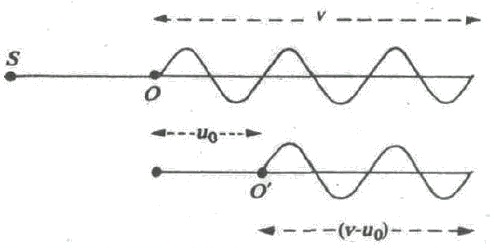If listener moves towards source, us is negative, and apparent frequency is provided by

f' = f(v + u0)/v

Source and Observer both in Motion:

When both source and observer are in motion (and approach each other). Source in motion causes the change in wavelength. Listener in motion results in the change of number of waves received. In such a case, apparent frequency f ' is given by

f' = length of block of waves received/reduced wavelength

= (v- u0)/(v - u0)

For electromagnetic waves, equation has to be altered. For sound, u0 and us are estimated relative to medium. This is due to medium determines wave speed. Though, E.M. waves don't need medium for propagation so that their speed relative to source or observer is always same. For these waves we have to consider only relative motion of source and observer. If u0 is speed of source relative to observer, and us << v, we can rewrite Equation as

f' = f(u/v - us)

= f(1 - us/v)-1

Using binomial expansion and retaining only first order terms in (us/uv) , we get

f' = f(1 + us/v)

In air navigation, we take us to be twice approach velocity of airplane. This is due to radar detects E.M. waves sent by it and reflected back by airplane.

Shock waves:

It is a thin transitive area propagating with supersonic speed in which there is quick increase of density, pressure and speeds of substance. Shock waves arise at explosions, detonation, supersonic movements of bodies, great electric discharges etc.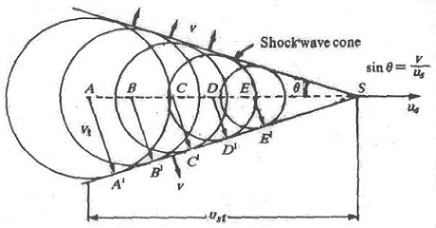Let us assume that source is at point A at t = 0. After time t, waves emitted at A are on sphere of radius vt. Since us>v, the distance travelled by source AS = ust is more than distance travelled by sound waves. Waves emitted at successive points, B, C, D, E.... are on line A'S, where circles are most crowded. We therefore see that sound waves pile up on the cone whose half angle is given by sinθ = v/us as shown in figure given above. No sound waves are present outside the cone. Velocity of sound waves is normal to surface of cone. When this cone hits the observer, he identifies abrupt arrival of large amplitude wave, called as the shock wave. The supersonic aircraft produces shock waves, also known as sonic booms, because of formation of two principal shock fronts; one at its nose and other at its tail. The strong boom can break window glasses or cause other harm to buildings. Shock waves are also produced in the ripple tank by the moving source for Mach numbers greater than one. Shock waves are produced by the boat moving faster than speed of water waves.

Tutorsglobe: A way to secure high grade in your curriculum (Online Tutoring)

Expand your confidence, grow study skills and improve your grades.

Since 2009, Tutorsglobe has proactively helped millions of students to get better grades in school, college or university and score well in competitive tests with live, one-on-one online tutoring.

Using an advanced developed tutoring system providing little or no wait time, the students are connected on-demand with a tutor at www.tutorsglobe.com. Students work one-on-one, in real-time with a tutor, communicating and studying using a virtual whiteboard technology.  Scientific and mathematical notation, symbols, geometric figures, graphing and freehand drawing can be rendered quickly and easily in the advanced whiteboard.

Free to know our price and packages for online physics tutoring. Chat with us or submit request at info@tutorsglobe.com